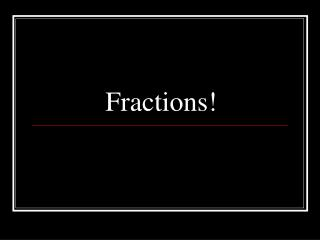DownloadDownload PresentationFractions!

# Fractions!

Télécharger la présentation## Fractions!

- - - - - - - - - - - - - - - - - - - - - - - - - - - E N D - - - - - - - - - - - - - - - - - - - - - - - - - - -
##### Presentation Transcript

1. Fractions!

2. Fractions A FRACTION is a number that represents a part of a whole. Fractions are useful whenever you need to split things up. 1 Whole Cake 4 pieces are the same as 1 whole cake

3. Parts of a Fraction 3 = the number of parts 4 = the total number of parts that equal a whole

4. Parts of a Fraction 3 = numerator 4 = denominator

5. Which number is circled? 3 = numerator 4

6. Look at this fraction: 3 5 3 5 of the scarf is blue.

7. ¾ looks like 1/4 1/4 3 4 1/4

8. Everyday Fractions Fractions are useful when you want to split something fairly with a friend.

9. Journal Question: Hayden and his friends cut a sub sandwich into 10 equal parts. He and his friends ate 7 parts. What fraction was eaten? *Draw a picture of the sub. Shade in the parts that were eaten. Write the fraction of the parts that were eaten.

10. FractionsParts of a Group Mrs. Willis

11. Essential Question: • How can I read and write fractions as part of a group?

12. Parts of a Group • Megan bought some buttons at the craft store. What fraction of Megan’s buttons are red? Megan’s Buttons: 2 Part Whole/ Total 8

13. Essential Question • How can I read and write Fractions as a part of a group?

14. Journal Question • Amaya has 12 ribbons. 1/ 12 of the ribbons are red and 3/ 12 of the ribbons are blue. The rest are yellow. What fraction of the ribbons are yellow? • DRAW A PICTURE OF THE RIBBONS!

16. Essential Question: How do I add Fractions? What are like Fractions?

17. Review: What is a fraction? Numerator Part Whole Denominator A FRACTION is a number that represents a part of a whole.

18. What is wrong with the problem? Jedda has 36 stickers. Tristen has 44 apples. How many more apples does Tristen have than Jedda?

19. Like Fractions… Fractions that have the same denominator are like fractions. In other words, fractions that have the same ______ are like fractions.

20. Adding Fractions A fruit punch recipe says to add 2/4 cup orange juice and 1/4 cup pineapple juice. How much juice is needed altogether?

21. Questions… Why doesn’t the denominator change? How could you use fraction bars to find 1/3 + 1/3?

22. Try some! 3/6 + 1/6 3/5 + 2/5

23. Word Problem Kayla has 6 plums and 2 apples. She buys two more apples. What fraction of the fruit are apples?

24. Essential Question: How do I add Fractions? What are like Fractions?

25. Journal Question Emory and Mykel share a pizza. Emory ate 3/8 of the pizza and Mykel ate 4/8 of the pizza. How much total pizza was eaten? *Draw a picture/ Use fraction blocks!

26. Subtracting Fractions Mrs. Willis

27. Essential Question What are like Fractions? How can I subtract Fractions?

28. Review: What is a fraction? Numerator Part Whole Denominator A FRACTION is a number that represents a part of a whole.

29. Like Fractions Find the Like Fractions: 1/4 2/3 4/5 2/4 3/6 2/5 1/8 2/ 8

30. Subtracting Fractions Review: How do I add fractions? 3/ 4 + 1/ 4= 2/9 + 4/9=

31. Problem • Nadia’s dad has 7/8 of his pan of corn bread to share with the family. If Nadia eats 2/8 of the corn bread, how much of the corn bread is left?

32. Try some! 3/6 – 1/6 5/8 - 2/8 7/10 – 4/10 4/5 – 1/5

33. Essential Question What are like Fractions? How can I subtract Fractions?

34. Problem Solving • Amaya has a pizza that is cut into 8 equal slices. Amaya ate 3/8 of the pizza. How much of the pizza is left?

35. Journal Question • Hayden subtracted 1/5 from 4/5 and got 3/0. Aundracia subtracted the two fractions and got 3/5. Who is right, Hayden or Aundracia? • SHOW WORK!!!

36. Fractions Mrs. Willis

37. Essential Question What is a Fraction?

38. Fractions Using your decimal models, show 6/10.